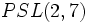# PSL(2,7) is isomorphic to PSL(3,2)

(diff) ← Older revision | Latest revision (diff) | Newer revision → (diff)$PSL(3,2) \cong PGL(3,2) \cong SL(3,2) \cong GL(3,2)$
Here, PSL(3,2) stands for the projective special linear group of degree three over field:F2.$PGL$ stands for the projective general linear group of degree three,$SL$ stands for the special linear group of degree three, and$GL$ stands for the general linear group of degree three.
The claim is that all of these isomorphic groups are isomorphic to the projective special linear group of degree two over field:F7, denoted$PSL(2,7)$.